# Powers And Roots Worksheet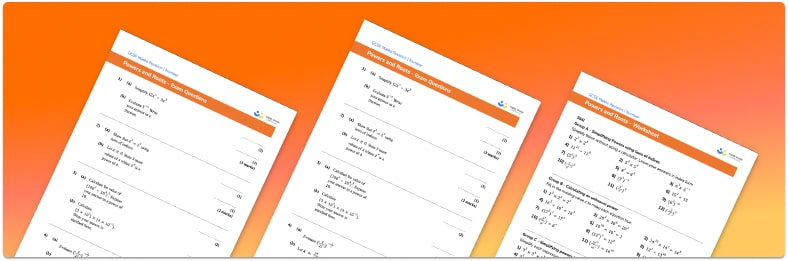• Section 1 of the powers and roots worksheet contains 36 skills-based powers and roots questions, in 3 groups to support differentiation
• Section 2 contains 4 applied powers and roots questions with a mix of worded problems and deeper problem solving questions
• Section 3 contains 4 foundation and higher level GCSE exam style powers and roots  questions
• Answers and a mark scheme for all powers and roots questions are provided
• Questions follow variation theory with plenty of opportunities for students to work independently at their own level
• All questions created by fully qualified expert secondary maths teachers
• Suitable for GCSE maths revision for AQA, OCR and Edexcel exam boards

• This field is for validation purposes and should be left unchanged.

You can unsubscribe at any time (each email we send will contain an easy way to unsubscribe). To find out more about how we use your data, see our privacy policy.

### Powers and roots at a glance

Powers, or exponents, are numbers written in superscript and denote repeated multiplication. The simplest case is square numbers where a superscript 2, this shows that a number is multiplied by itself. For cube numbers, with a superscript 3, the multiplication is carried out three times and so on for any integer (whole number) power.

Rooting is the inverse operation. The square root is the inverse of squaring, the cube root is the inverse of cubing, and so on. The root finds the original number that was multiplied.

The laws of indices extend these ideas to explain what happens when we multiply, divide or carry out other operations on expressions containing powers, both numeric and algebraic. We can extend these ideas further to using negative numbers or fractions as powers, and estimating powers and roots using known facts.

Looking forward, students can then progress to additional number worksheets, for example a or aFor more teaching and learning support on Number our GCSE maths lessons provide step by step support for all GCSE maths concepts.

## Related worksheets

Squares, Cubes, And Roots Worksheet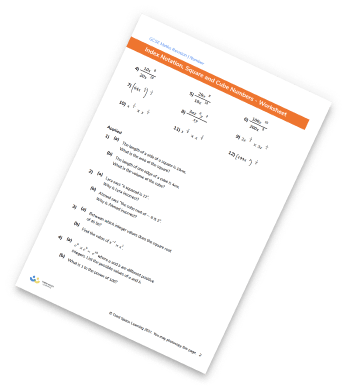Rational And Irrational Numbers Worksheet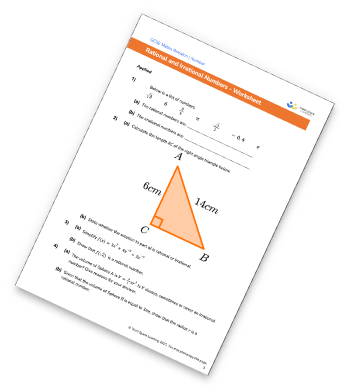Surds Worksheet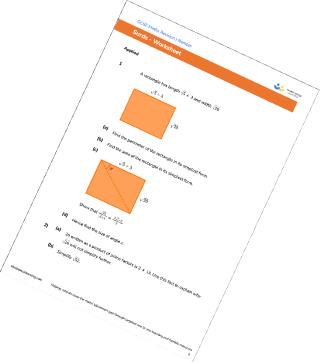Multiplying And Dividing Standard Form Worksheet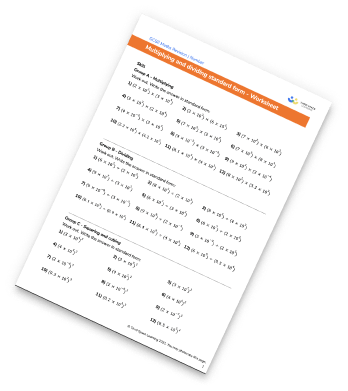## Do you have KS4 students who need more focused attention to succeed at GCSE?There will be students in your class who require individual attention to help them succeed in their maths GCSEs. In a class of 30, it’s not always easy to provide.

Help your students feel confident with exam-style questions and the strategies they’ll need to answer them correctly with our dedicated GCSE maths revision programme.

Lessons are selected to provide support where each student needs it most, and specially-trained GCSE maths tutors adapt the pitch and pace of each lesson. This ensures a personalised revision programme that raises grades and boosts confidence.

Find out more